# Pearl No.1 - The Min Missing Natural NumberProf. Richard Simpson Bird is a Supernumerary Fellow of Computation at Lincoln College, Oxford, England, and former director of the Oxford University Computing Laboratory. His research interests include:

• The algebra of programming
• The calculation of algorithms from their specification
• Functional programming
• Algorithm design

In 2010, he wrote the book Pearls of Functional Algorithm Design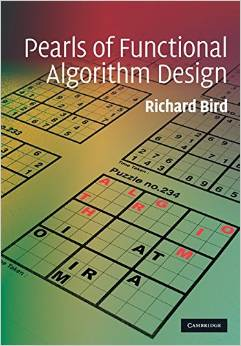This book presents 30 algorithm problems and their functional solutions. The reason that they are called as pearls is what I quote below:

Just as natural pearls grow from grains of sand that have irritated oysters, these programming pearls have grown from real problems that have irritated programmers. The programs are fun, and they teach important programming techniques and fundamental design principles.

These pearls are all classic and togehter with the well presented functional approaches, they become very helpful for ones who really wish to sharpen their functional programming on algorithms. I have so far solved / fully understood 17 pearls and hmm...the rest are indeed difficult, at least for me. Nevertheless, I would like to share my journey of studying this book with you via a series of posts, each of which is a pearl in the book. All the posts will differ from the book in the following ways:

1. Only OCaml is used for the implementations (for the obvious reason: I am a fan of OCaml)
2. More general (functional) analysis techniques are adopted. The book heavily focuses on algebraic approach, namely, design by calculation, which honestly is too much for me. I mean I can understand the methodology, but cannot master it. So during my study, I did not replicate the algebraic thinking; instead, I tried to sniff around the functional sprit behind the curtain and consider the algebraic part as the source of hints.
3. Tail-recursive implementation will be provided if possible. The haskell implementations in the book do not consider much about tail-recursive because a) haskell is lazy; b) haskell's compiler is doing clever things to help recursion not blow. OCaml is different. It is not lazy and does not rely on the compiler to do optimisations on the potential stackoverflow. So as OCaml developers, we need to care about tail-recursive explicitly.

Ok. Let's get started.

# The Min Missing Natural Number

Given a unordered list of distinct natural numbers, find out the minimum natural number that is not in the list.

For example, if the list is [8; 2; 3; 0; 12; 4; 1; 6], then 5 is the minimum natural number that is missing.

O(n) solution is desired.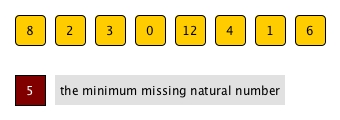# Analysis of the problem description

The description of an algorithm problem specifies the input, the output and the constraint. Yet, it is more than just telling us what to achieve. Most of the time, the literatures can provide us hints for possible solutions. Let's break down the description first:

1. unordered list
2. distinct
3. natural numbers
4. minimum and missing

### unordered list

The input list is not sorted. If this is specified explicitly, it implies that ordering is important here. In other words, if the list was sorted, then the problem would not be a problem any more or at least much easier to solve.

### distinct

There are no duplicates inside.

### natural numbers

All numbers are non-negative integers, i.e., 0, 1, 2, ... This puts a lower boundary on the possible numbers in the input list.

### minimum and missing

There might be unlimited numbers not in the input list, but we just need to find the smallest one. When our goal is to locate something with certain characteristic, it would normally be a selection problem. Moreover, for problems related min or max, they normally can be solved by somehow bringing sorting; however, sorting will heavily involve moving all numbers around. As our target is only one number, sorting can be an overkill.

# An easy but not optimal solution

From the analysis above, it is obvious that sorting can solve our problem quite easily. We can simply

1. Sort the input list
2. If the first number is not 0, then the result is 0
3. Scan every consecutive pair (x, y)
4. If y - x > 1 then the result is x + 1
5. If point 4 never happens, then the result is the last number plus 1.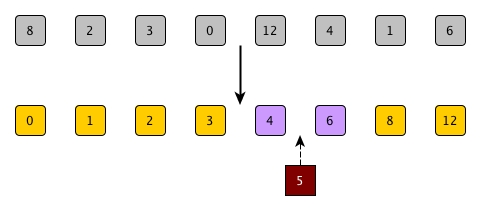let min_missing_trivial l =
let sl = List.sort compare l in
let rec find = function
| [] -> None
| x::[] -> Some (x+1)
| x::y::_ when y - x > 1 -> Some (x+1)
| _::tl -> find tl
in
(* adding -1 can cover point 2 and make find work for all *)
find ((-1)::sl)


Have we solved the problem? Let's have a look. The solution above can achieve the goal indeed. However, the time complexity of this solution is O(nlogn) since the sorting bit is dominating, which is worse than the required O(n).

We have to try harder.

# Do we need complete order?

So, if we do a sorting, we can obtain the answer but it is too slow. Let's have a look again at what we get after sorting.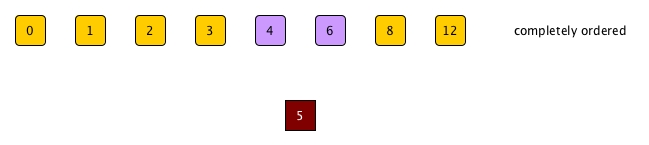If the list is sorted, then it provides us a chance where we check the consecutiveness of the numbers and the first gap is what we want. There are two questions though:

Q1. Shall we care about the consecutiveness after 6?

The answer is no. Since we are chasing for the minimum, i.e., the first missing one, the order of the numbers after the gap doesn't matter any more.

For example, even if 12 is before 8, the result won't be affected.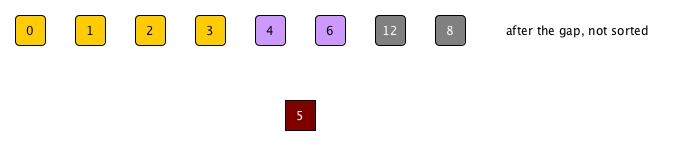Q2. Is the order of all the numbers before the gap important?

Let's randomly mess up the order of 0, 1, 2, and 3 a little: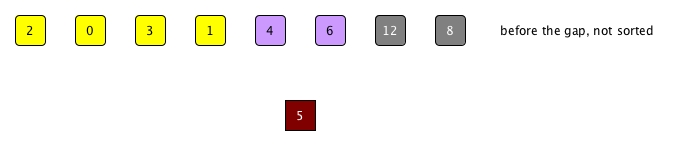It seems fine as the messed order of those 4 numbers does not affect the position of 4 and 6. But hang on a minute, something is not right there.

We replied on the consecutiveness of 0, 1, 2, and 3 to locate the first gap, and the consecutiveness can be checked via the numbers being sorted. Hence, if the order before the gap was not maintained, how could we scan for consecutiveness and find the gap in the first place? It sounds like a chicken and egg thing.

So can we check for the consecutiveness of the numbers without sorting them?

# Hints hidden in "distinct natural numbers"

Yes, we can, and now it is the time to ask for help from distinct natural numbers.

As we described before, natural numbers are integers euqal to or larger than 0. This lower bound 0 plus the constraint of no duplicates gives us the opportunity to check for consecutiveness without requiring all numbers being sorted. Let's first see a perfect consecutive sequnce (starting from 0) of natural numbers: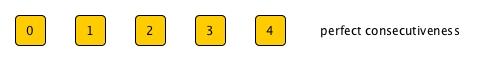They are sorted of course. Is there any other characteristic? Or say, for a number inside the sequence, how many other numbers are less than it (on its left side)?For number 4, there will exact 4 natural numbers less than itself. The same thing will apply on any numbers as long as all those belong to a perfect consecutive sequence starting from 0.

This is also a two-way mapping, i.e., if we are told that there are 4 numbers less than 4 and all of them are before 4, we can be sure that all five numbers can form a consecutive sequence. Most importantly, now whether all numbers are in order or not does not matter any more.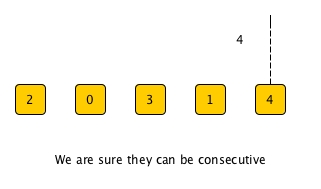What does a perfect consecutiveness imply?

It implies that among the sequence, no one is missing and if anyone is missing, it must be on the right side of the max of the sequence.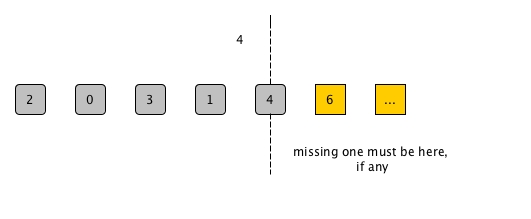What if for a number, the number of smaller ones does not match its own value?

It means the sequence up to the number won't be consecutive, which implies that there must be at least one a natural number missing, right?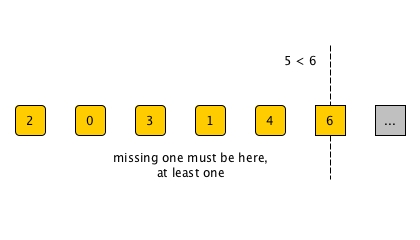Now we have the weapon we want. In order to check consecutiveness, or say, to know the region where the min missing natural number is, we don't need complete sorting any more. Instead, we just to

1. pick a number x from the sequence
2. put all other smaller numbers to its left and count how many are those in num_less
3. put all larger ones to its right
4. If num_less = x, then it means x's left branch is perfect and the missing one must be in its right branch. We repeat the whole process in the sequence of right hand side.
5. Otherwise, we repeat in the left branch. Note that due to distinct, it is impossible num_less > x.

In this way, we cannot identify the min missing one in one go, but we can narrow down the range where it might be through each iteration and eventually we will find it when no range can be narrowed any more.

Sounds like a good plan? let's try to implement it.

# Partition and Implementation

Do you feel familiar with the process we presented above? It is actually a classic partition step which is the key part of quicksort we talked about.

Of course, in this problem, it won't be a standard partition as we need to record num_less. The code should be easy enough to write:

let partition x l =
let f (num_less, left, right) y =
if y < x then num_less+1, y::left, right
else num_less, left, y::right
in
List.fold_left f (0, [], []) l


Also our solver function should be straightword too:

let min_missing l =
let rec find lo = function
| [] -> lo
| x::tl ->
let num_less, left, right = partition x tl in
if num_less + lo = x then find (x+1) right
else find lo left
in
find 0 l

(* Why do we introduce lo?
I will leave this question to the readers *)


## Time complexity of our new solution

For the very 1st iteration, we need to scan all numbers, so costs O(n) for this step. But we can skip out ideally half of the numbers.

So for the 2nd iteration, it costs O(n*(1/2)). Again, further half will be ruled out.

...

So the total cost would be O(n * (1 + 1/2 + 1/4 + 1/8 + ...)) = O(n * 2) = O(n).